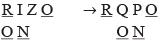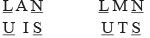Courses

# Olympiad Test: Alphabet Test -1

## 15 Questions MCQ Test Science Olympiad Class 7 | Olympiad Test: Alphabet Test -1

Description
This mock test of Olympiad Test: Alphabet Test -1 for Class 7 helps you for every Class 7 entrance exam. This contains 15 Multiple Choice Questions for Class 7 Olympiad Test: Alphabet Test -1 (mcq) to study with solutions a complete question bank. The solved questions answers in this Olympiad Test: Alphabet Test -1 quiz give you a good mix of easy questions and tough questions. Class 7 students definitely take this Olympiad Test: Alphabet Test -1 exercise for a better result in the exam. You can find other Olympiad Test: Alphabet Test -1 extra questions, long questions & short questions for Class 7 on EduRev as well by searching above.
QUESTION: 1

### Arrange the given words in alphabetical order and choose the one that comes first.

Solution:

waiting, wanting, watching, waving.

QUESTION: 2

### Arrange the given words in alphabetical order and choose the one that comes first.

Solution:

Lapse, Laurel, Leave, Leisure.

QUESTION: 3

### Arrange the given words in alphabetical order and choose the one that comes first.

Solution:

Project, Property, Protein, Proverb.

QUESTION: 4

Arrange the given words in alphabetical order and choose the one that comes first.

Solution:

Dissect, Dissipate, Dissociate, Distract.

QUESTION: 5

Arrange the given words in alphabetical order and choose the one that comes first.

Solution:

Pagan, Page, Pageant, Palate.

QUESTION: 6

Arrange the given words in alphabetical order and tick the one that comes last.

Solution:

Mink, Murder, Murmer, Music.

QUESTION: 7

Arrange the given words in alphabetical order and tick the one that comes last.

Solution:

Marigold, Marine, Marital, Maritime.

QUESTION: 8

Arrange the given words in alphabetical order and tick the one that comes last.

Solution:

QUESTION: 9

Arrange the given words in alphabetical order and tick the one that comes last.

Solution:

Latent, Latitude, Lattice, Launch.

QUESTION: 10

Arrange the given words in alphabetical order and tick the one that comes last.

Solution:

QUESTION: 11

Directions: In each of the following questions find out how many such pairs of letters are there in the given word each of which has as many letters between them in the word as in the English alphabet.

Solution:

PARARADISE → P Q R A B C D

QUESTION: 12

​Directions: In each of the following questions find out how many such pairs of letters are there in the given word each of which has as many letters between them in the word as in the English alphabet.

HORIZON

Solution:QUESTION: 13

​Directions: In each of the following questions find out how many such pairs of letters are there in the given word each of which has as many letters between them in the word as in the English alphabet.

LANGUISH

Solution:QUESTION: 14

​Directions: In each of the following questions find out how many such pairs of letters are there in the given word each of which has as many letters between them in the word as in the English alphabet.

Q. Which letter in word CYBERNETICS occupies the same position as it does in the English alphabet?

Solution:

I is the 9th letter in the word as well as in the english alphabet.

QUESTION: 15

​Directions: In each of the following questions find out how many such pairs of letters are there in the given word each of which has as many letters between them in the word as in the English alphabet.

HACKLE

Solution:

H A C K        H I J K
A C K L E     A  B C D E
K L                       K L